Created December 15, 2020 © CC BY-NC-ND

# Digital Blood Pressure Monitor

Detecting blood pressure using Korotkoff sounds

AdvancedFull instructions provided24 hours264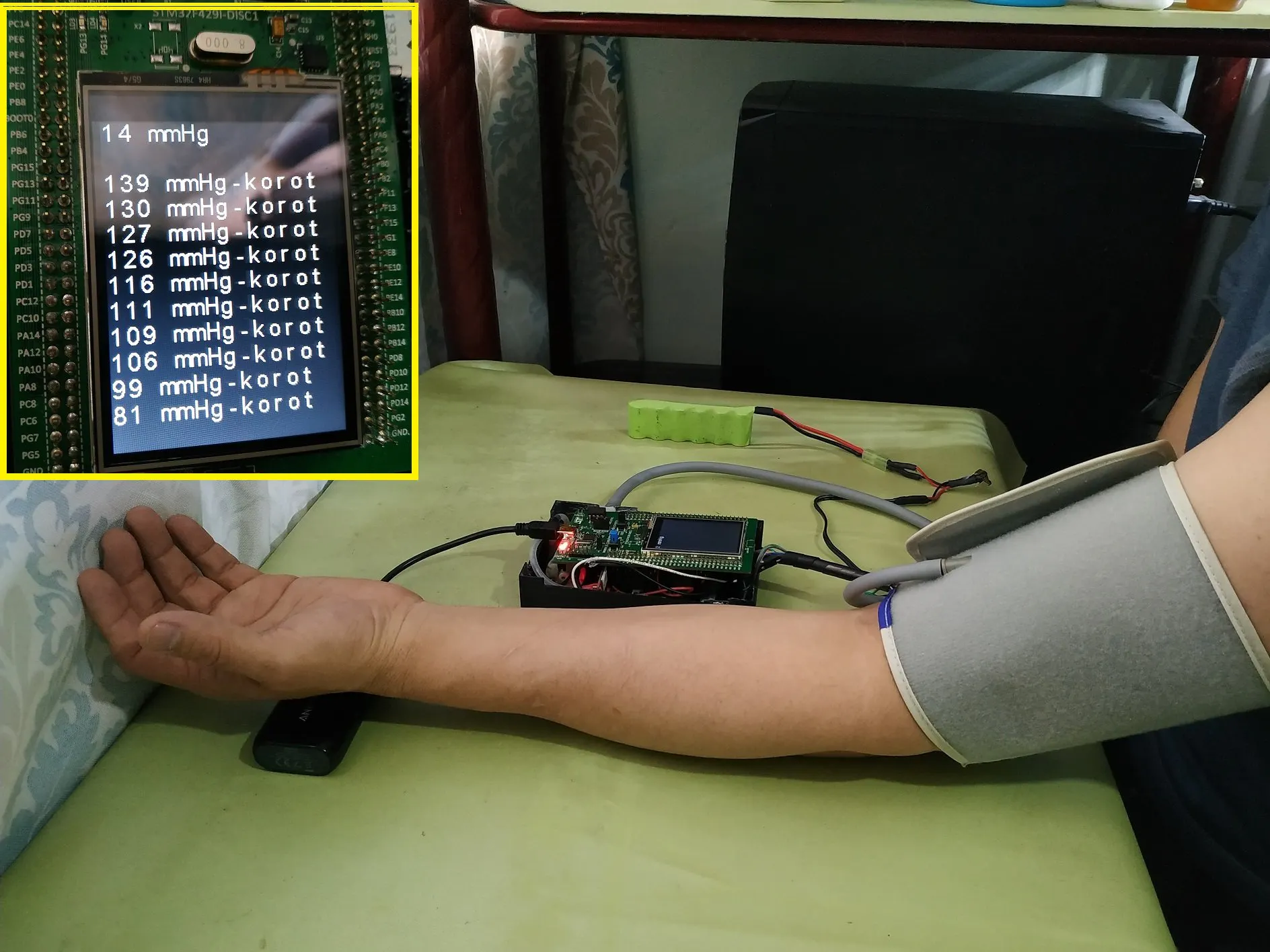## Things used in this project

### Hardware components

 STM32F429I-DISC1
×1
 ESP32-WROOM-32
×1
 SparkFun - Micropressure Sensor
×1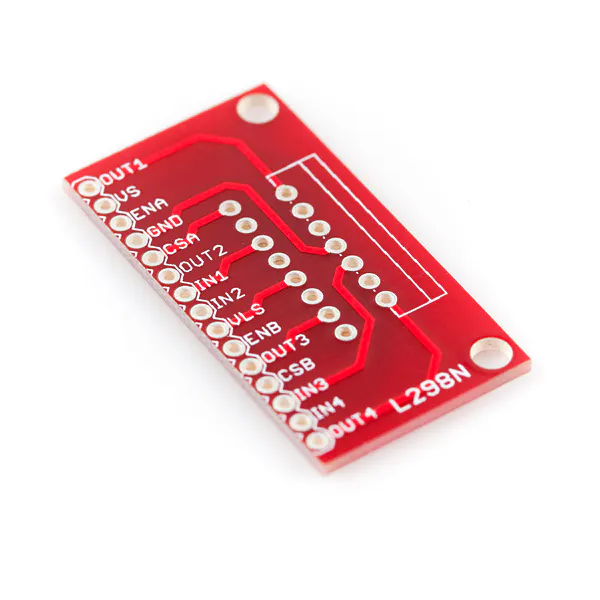SparkFun Full-Bridge Motor Driver Breakout - L298N
×1
 Air Pump
×1
 Pneumatic Solenoid Valve
×1
 Air Release Valve
×1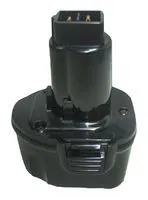Rechargeable Battery, 7.2 V
×1

### Software apps and online servicesArduino IDE

### Hand tools and fabrication machines

 Arm Cuff Blood Pressure Monitor3D Printer (generic)
 Aneroid gauge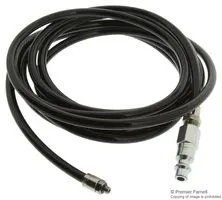ESD Air Hose, with Standard FittingHot glue gun (generic)

## Schematics

### Digital Blood Pressure Monitor

SCHEMATIC DIAGRAM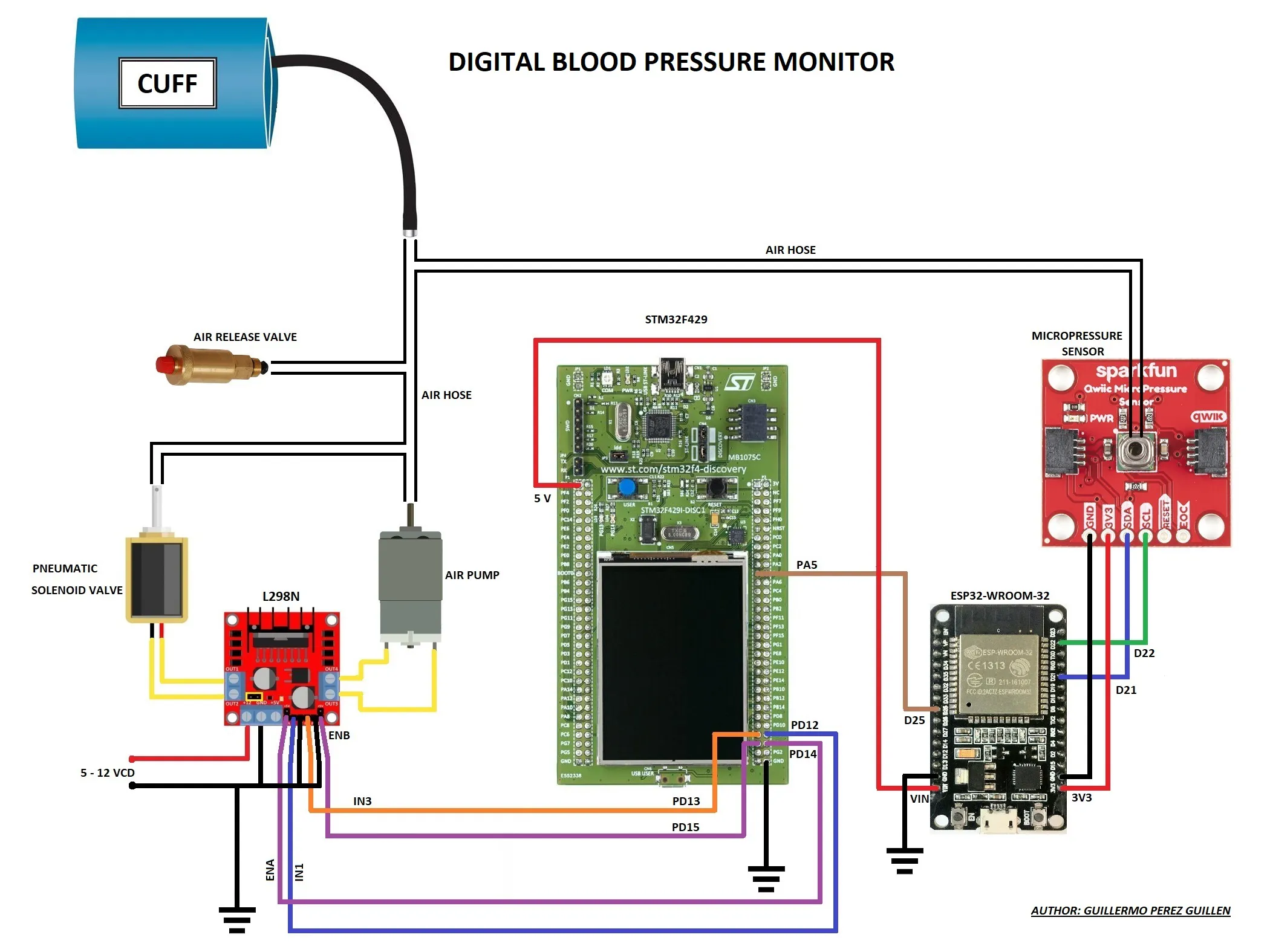### Digital Blood Pressure Monitor

FLOWCHART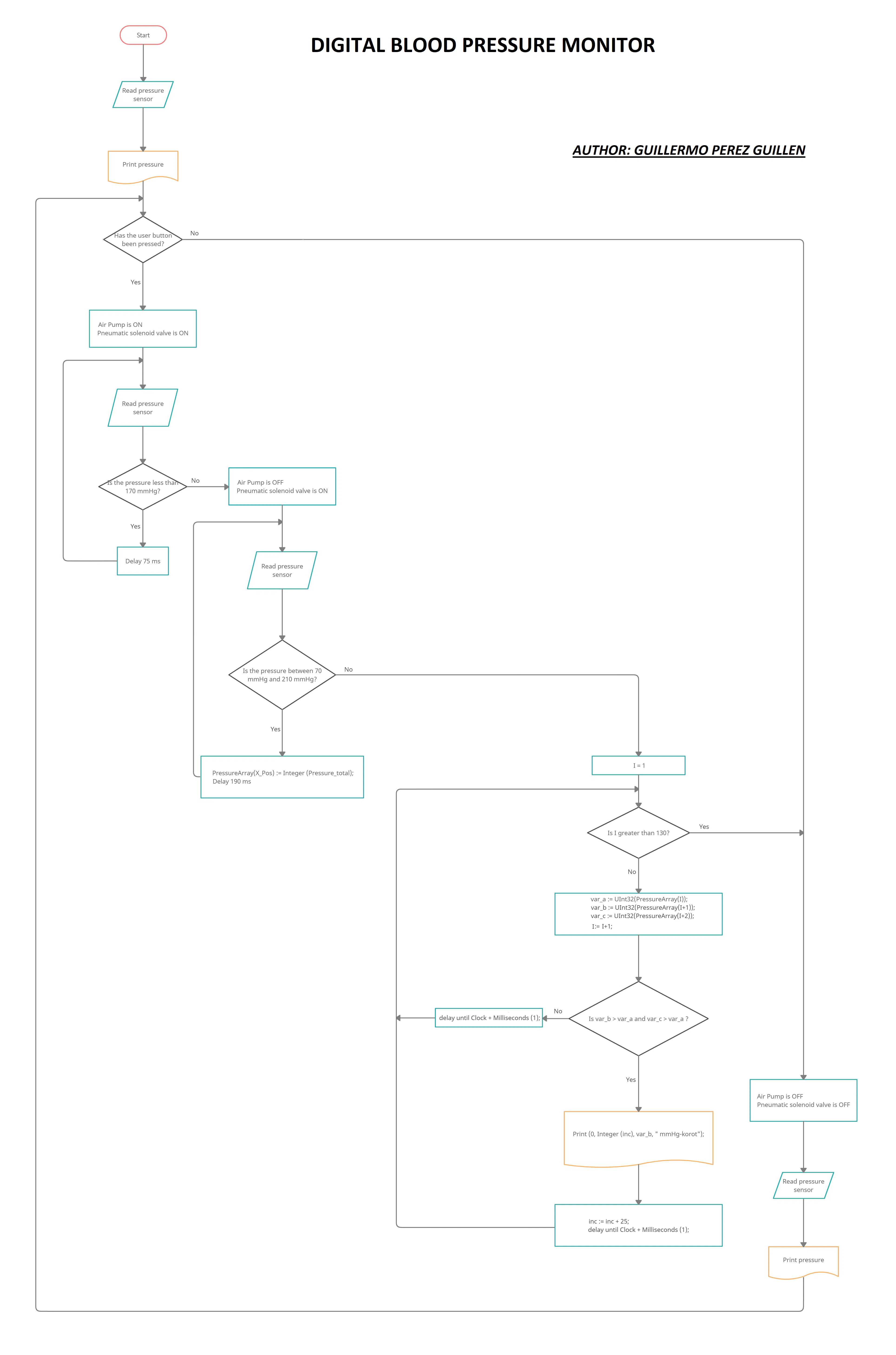### STL FILES

Box, Cover and Support

## Code

Developed with GNAT Programming Studio. This code must be compiled and uploaded to the STM32F429I board
```--  AUTHOR: GUILLERMO ALBERTO PEREZ GUILLEN

with Last_Chance_Handler;  pragma Unreferenced (Last_Chance_Handler);

with STM32.Device;  use STM32.Device;
with HAL;           use HAL;
with STM32.GPIO;    use STM32.GPIO;

with STM32.User_Button; --PA0 button
with LCD_Std_Out;

procedure Digital_Blood_Pressure_Monitor is

type Integer_Array is array (Integer range <>) of Integer with Default_Component_Value => 0;
PressureArray : Integer_Array (0..200);

Converter     : Analog_To_Digital_Converter renames ADC_1; -- ADC_1
Input_Channel : constant Analog_Input_Channel := 5; --5
Input         : constant GPIO_Point := PA5;   -- PA5 analog input

Solenoid_valve  : GPIO_Point renames PD12; -- solenoid valve
Motor           : GPIO_Point renames PD13; -- air pump
Enable_a        : GPIO_Point renames PD14;
Enable_b        : GPIO_Point renames PD15;

Ports : constant GPIO_Points := (Solenoid_valve, Motor, Enable_a, Enable_b);

All_Regular_Conversions : constant Regular_Channel_Conversions :=
(1 => (Channel => Input_Channel, Sample_Time => Sample_144_Cycles));

Raw : UInt32 := 0;
Volts : UInt32 := 0;
Pressure : UInt32 := 0;
Pressure_total : UInt32 := 0;
X_Pos: Integer := 0;
--PressureAddr : UInt32 := 1; -- No such thing as a zero Pressure address
var_a : UInt32 := 0;
var_b : UInt32 := 0;
var_c : UInt32 := 0;
inc : UInt32 := 50;

Successful : Boolean;

procedure Initialize_Ports;

procedure Print (X, Y : Natural; Value : UInt32; Suffix : String := "");

procedure Configure_Analog_Input;

----------------------------
-- Initialize_Ports        --
----------------------------

procedure Initialize_Ports is
begin
Enable_Clock (GPIO_D);

Configure_IO
(Ports,
(Mode        => Mode_Out,
Resistors   => Floating,
Speed       => Speed_100MHz,
Output_Type => Push_Pull));
end Initialize_Ports;

-----------
-- Print --
-----------

procedure Print (X, Y : Natural; Value : UInt32; Suffix : String := "") is
Value_Image : constant String := Value'Img;
begin
LCD_Std_Out.Put (X, Y, Value_Image (2 .. Value_Image'Last) & Suffix & "   ");
end Print;

----------------------------
-- Configure_Analog_Input --
----------------------------

procedure Configure_Analog_Input is
begin
Enable_Clock (Input);
Configure_IO (Input, (Mode => Mode_Analog, Resistors => Floating));
end Configure_Analog_Input;

begin
Initialize_Ports;
STM32.User_Button.Initialize;
Solenoid_valve.Clear;
Motor.Clear;
Enable_a.Clear;
Enable_b.Clear;

Configure_Analog_Input;

Enable_Clock (Converter);

Configure_Common_Properties
(Mode           => Independent,
Prescalar      => PCLK2_Div_2,
DMA_Mode       => Disabled,
Sampling_Delay => Sampling_Delay_5_Cycles);

Configure_Unit
(Converter,
Alignment  => Right_Aligned);

Configure_Regular_Conversions
(Converter,
Continuous  => False,
Trigger     => Software_Triggered,
Enable_EOC  => True,
Conversions => All_Regular_Conversions);

Enable (Converter);

loop

Start_Conversion (Converter);
Poll_For_Status (Converter, Regular_Channel_Conversion_Complete, Successful);

Solenoid_valve.Clear;
Motor.Clear;
Enable_a.Clear;
Enable_b.Clear;

Raw := UInt32 (Conversion_Value (Converter));
Volts := UInt32  ((Float (Raw) / 4096.0) * 3000.0);   -- 4096 ADC = 3000 mV
Pressure := UInt32 ((Float (Volts) / 3000.0) * 255.0);   -- 3000 mV = 255 mmHg
Pressure_total := UInt32 (float (Pressure) - 8.0);   -- 3000 mV = 255 mmHg
Print (0, 0, Pressure_total, " mmHg"); -- print blood pressure

if STM32.User_Button.Has_Been_Pressed then -- Btn pressed then go to 170 mmHg
Start_Conversion (Converter);
Poll_For_Status (Converter, Regular_Channel_Conversion_Complete, Successful);
Solenoid_valve.Set;   -- solenoid valve is ON
Motor.Set;   -- air pump is ON
Enable_a.Set;
Enable_b.Set;
Raw := UInt32 (Conversion_Value (Converter));
Volts := UInt32  ((Float (Raw) / 4096.0) * 3000.0);   -- 4096 ADC = 3000 mV
Pressure := UInt32 ((Float (Volts) / 3000.0) * 255.0);   -- 3000 mV = 255 mmHg
Pressure_total := UInt32 (float (Pressure) - 8.0);   -- 3000 mV = 255 mmHg
Print (0, 0, Pressure_total, " mmHg"); -- print blood pressure

while Pressure_total <= 170 loop
Start_Conversion (Converter);
Poll_For_Status (Converter, Regular_Channel_Conversion_Complete, Successful);
Raw := UInt32 (Conversion_Value (Converter));
Volts := UInt32  ((Float (Raw) / 4096.0) * 3000.0);   -- 4096 ADC = 3000 mV
Pressure := UInt32 ((Float (Volts) / 3000.0) * 255.0);   -- 3000 mV = 255 mmHg
Pressure_total := UInt32 (float (Pressure) - 8.0);   -- 3000 mV = 255 mmHg
Print (0, 0, Pressure_total, " mmHg"); -- print blood pressure
delay until Clock + Milliseconds (75);
end loop;

Solenoid_valve.Set;   -- solenoid valve is ON
Motor.Clear;   -- air pump is OFF
Enable_a.Set;
Enable_b.Clear;

delay until Clock + Milliseconds (100);

while Pressure_total > 70 and Pressure_total <= 210 loop
Start_Conversion (Converter);
Poll_For_Status (Converter, Regular_Channel_Conversion_Complete, Successful);
Raw := UInt32 (Conversion_Value (Converter));
Volts := UInt32  ((Float (Raw) / 4096.0) * 3000.0);   -- 4096 ADC = 3000 mV
Pressure := UInt32 ((Float (Volts) / 3000.0) * 255.0);   -- 3000 mV = 255 mmHg
Pressure_total := UInt32 (float (Pressure) - 8.0);   -- 3000 mV = 255 mmHg
Print (0, 0, Pressure_total, " mmHg"); -- print blood pressure

PressureArray(X_Pos) := Integer (Pressure_total);
X_Pos := X_Pos + 1;
delay until Clock + Milliseconds (190);
end loop;

--         for I in 1 .. 140 loop
--            PressureAddr := UInt32(PressureArray(I));
--            Print (0, 50, PressureAddr, " mmHg"); -- print blood pressure
--            delay until Clock + Milliseconds (50); -- 4 secs and inflating the cuff to 80 mmHg
--         end loop;

for I in 1 .. 130 loop
var_a := UInt32(PressureArray(I));
var_b := UInt32(PressureArray(I+1));
var_c := UInt32(PressureArray(I+2));

if var_b > var_a and var_c > var_a then

Print (0, Integer (inc), var_b, " mmHg-korot");
inc := inc + 25;
delay until Clock + Milliseconds (1);

else

delay until Clock + Milliseconds (1);

end if;

end loop;

else
Start_Conversion (Converter);
Poll_For_Status (Converter, Regular_Channel_Conversion_Complete, Successful);
Solenoid_valve.Clear; -- valve is OFF
Motor.Clear; -- stop the motor
Enable_a.Clear;
Enable_b.Clear;
Raw := UInt32 (Conversion_Value (Converter));
Volts := UInt32  ((Float (Raw) / 4096.0) * 3000.0);   -- 4096 ADC = 3000 mV
Pressure := UInt32 ((Float (Volts) / 3000.0) * 255.0);   -- 3000 mV = 255 mmHg
Pressure_total := UInt32 (float (Pressure) - 8.0);   -- 3000 mV = 255 mmHg
Print (0, 0, Pressure_total, " mmHg"); -- print blood pressure
delay until Clock + Milliseconds (100); -- delay to the next step

end if;
end loop;

end Digital_Blood_Pressure_Monitor;
```

### digital_blood_pressure_monitor.gpr

Project developed with GNAT Programming Studio
```with "../../../../../boards/stm32f429_discovery/stm32f429_discovery_full.gpr";

project Digital_Blood_Pressure_Monitor extends "../../../../../examples/shared/common/common.gpr" is
for Source_Dirs use ("src");
for Object_Dir use "obj" & STM32F429_Discovery_Full.Build;
for Main use ("digital_blood_pressure_monitor.adb");
for Languages use ("Ada");
for Create_Missing_Dirs use "true";
package Builder is
for Global_Configuration_Pragmas use "gnat.adc";
end Builder;
package Compiler renames STM32F429_Discovery_Full.Compiler;
end Digital_Blood_Pressure_Monitor;
```

### pressure_sensor

Arduino
This code must be uploaded to the ESP32-WROOM-32 board
```#include<Wire.h>
#include <SparkFun_MicroPressure.h>
SparkFun_MicroPressure mpr; // Use default values with reset and EOC pins unused

#define DAC2 26

float min_pressure = 600;

void setup() {
// Initalize UART, I2C bus, and connect to the micropressure sensor
Serial.begin(9600);
Wire.begin();
if(!mpr.begin())
{
Serial.println("Cannot connect to MicroPressure sensor.");
while(1);
}
}

void loop() {
float sensor_pressure = (mpr.readPressure(INHG)*25.4);

if(sensor_pressure<min_pressure){
Serial.println("new minimum pressure");
min_pressure=sensor_pressure;
}

int pressure_total = sensor_pressure - min_pressure;

dacWrite(DAC2, pressure_total);
Serial.print(pressure_total);
Serial.println(";");
delay(200);
}
```

### GITHUB REPOSITORY CODE

Digital Blood Pressure Monitor Code

## Credits

### Guillermo Perez Guillen

45 projects • 51 followers
Electronics and Communications Engineer (ECE): Ten awards in Hackster / Finalist in 2021 Hackaday Prize / Two runner up winner in element14